# logKDE: log-transformed kernel density estimationThe goal of logKDE is to provide a set of functions for kernel density estimation on the positive domain, using log-kernel density functions, for the R programming environment. The main functions of the package are the `logdensity` and `logdensity_fft` functions. The choice of functional syntax was made to resemble those of the `density` function, for conducting kernel density estimation on the real domain. The `logdensity` function conducts density estimation, via first principle computations, whereas `logdensity_fft` utilizes fast-Fourier transformation in order to speed up computation. The use of `Rcpp` guarantees that both methods are sufficiently fast for large data scenarios.

Currently, a variety of kernel functions and plugin bandwidth methods are available. By default both `logdensity` and `logdensity_fft` are set to use log-normal kernel functions (`kernel = 'gaussian'`) and Silverman’s rule-of-thumb bandwidth, applied to log-transformed data (`bw = 'nrd0'`). However, the following kernels are also available:

• log-Epanechnikov (`kernel = 'epanechnikov'`),
• log-Laplace (`kernel = 'laplace'`),
• log-logistic (`kernel = 'logistic'`),
• log-triangular (`kernel = 'triangular'`),
• log-uniform (`kernel = 'uniform'`).

The following plugin bandwidth methods are also available:

• all of the methods that available for density, applied to log-transformed data (see `?bw.nrd` regarding the options),
• unbiased cross-validated bandwidths in the positive domain (`bw = 'logcv'`),
• a Silverman-type rule-of-thumb that optimizes the kernel density estimator fit, compared to a log-normal density function (`bw = 'logg'`).

The `logdensity` and `logdensity_fft` functions also behave in the same way as `density`, when called within the `plot` function. The usual assortment of commands that apply to `plot` output objects can also be called.

For a comprehensive review of the literature on positive-domain kernel density estimation, thorough descriptions of the mathematics relating to the methods that have been described, simulation results, and example applications of the `logKDE` package, please consult the package vignette. The vignette is available via the command `vignette('logKDE')`, once the package is installed.

## Installation

If `devtools` has already been installed, then the most current build of `logKDE` can be obtained via the command:

``devtools::install_github('andrewthomasjones/logKDE',build_vignettes = T)``

The latest stable build of `logKDE` can be obtain from CRAN via the command:

``install.packages("logKDE", repos='http://cran.us.r-project.org')``

An archival build of `logKDE` is available at https://zenodo.org/record/1317784. Manual installation instructions can be found within the R installation and administration manual https://cran.r-project.org/doc/manuals/r-release/R-admin.html.

## Examples

### Example 1

In this example, we demonstrate that `logdensity` has nearly identical syntax to `density`. We also show that the format of the outputs are also nearly identical.

``````## Load 'logKDE' library.
library(logKDE)

## Set a random seed.
set.seed(1)

## Generate strictly positive data.
## Data are generated from a chi-squared distribution with 12 degrees of freedom.
x <- rchisq(100,6)

## Construct and print the output of the function 'density'.
density(x)
#>
#> Call:
#>  density.default(x = x)
#>
#> Data: x (100 obs.);  Bandwidth 'bw' = 1.018
#>
#>        x                y
#>  Min.   :-2.366   Min.   :0.0000475
#>  1st Qu.: 2.547   1st Qu.:0.0072263
#>  Median : 7.459   Median :0.0331904
#>  Mean   : 7.459   Mean   :0.0508396
#>  3rd Qu.:12.372   3rd Qu.:0.1013289
#>  Max.   :17.284   Max.   :0.1312107

## Construct and print the output of the function 'logdensity'.
logdensity(x)
#>
#> Call:
#>  logdensity(x = x)
#>
#> Data: x (100 obs.);  Bandwidth 'bw' = 0.1923
#>
#>        x                 y
#>  Min.   : 0.1111   Min.   :0.00000
#>  1st Qu.: 3.7851   1st Qu.:0.02313
#>  Median : 7.4592   Median :0.06527
#>  Mean   : 7.4592   Mean   :0.06707
#>  3rd Qu.:11.1333   3rd Qu.:0.11219
#>  Max.   :14.8073   Max.   :0.13698

## Plot the 'density' output object.
plot(density(x))``````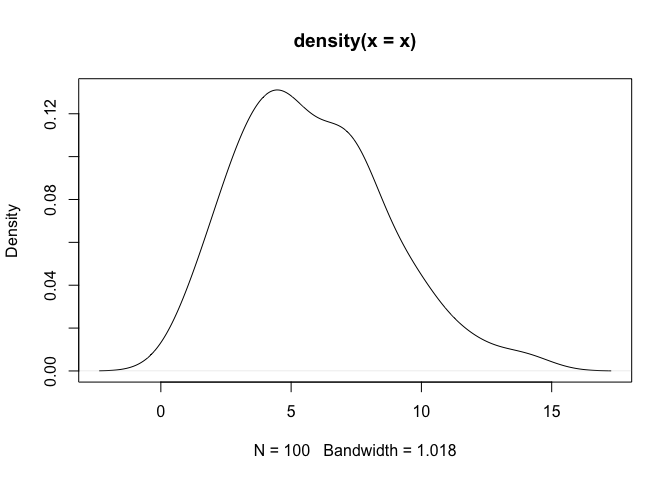``````
## Plot the 'logdensity' output object.
plot(logdensity(x))``````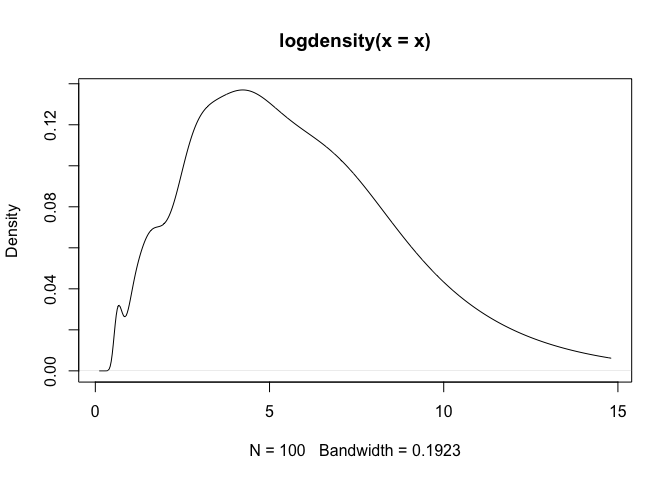As a note, one can observe that `density` assigns positive probability to negative values. Since we know that the chi-squared generative model generates only positive values, this is an undesirable result. The log-transformed kernel density estimator that is produced by `logdensity` only assigns positive probability to positive values, and is thus bona fide in this estimation scenario.

### Example 2

In this example, we showcase the variety of kernel functions that are available in the package. Here, log-transformed kernel density estimators are constructed using the `logdensity` function.

``````## Load 'logKDE' library.
library(logKDE)

## Set a random seed.
set.seed(1)

## Generate strictly positive data.
## Data are generated from a chi-squared distribution with 12 degrees of freedom.
x <- rchisq(100,12)

## Construct a log-KDE using the data, and using each of the available kernel functions.
logKDE1 <- logdensity(x,kernel = 'gaussian',from = 1e-6,to = 30)
logKDE2 <- logdensity(x,kernel = 'epanechnikov',from = 1e-6,to = 30)
logKDE3 <- logdensity(x,kernel = 'laplace',from = 1e-6,to = 30)
logKDE4 <- logdensity(x,kernel = 'logistic',from = 1e-6,to = 30)
logKDE5 <- logdensity(x,kernel = 'triangular',from = 1e-6,to = 30)
logKDE6 <- logdensity(x,kernel = 'uniform',from = 1e-6,to = 30)

## Plot the true probability density function of the generative model.
plot(c(0,30),c(0,0.1),type='n',xlab='x',ylab='Density',main='Example 2')
curve(dchisq(x,12),from = 0,to = 30,add = T)

## Plot each of the log-KDE functions, each in a different rainbow() colour.
lines(logKDE1\$x,logKDE1\$y,col = rainbow(7))
lines(logKDE2\$x,logKDE2\$y,col = rainbow(7))
lines(logKDE3\$x,logKDE3\$y,col = rainbow(7))
lines(logKDE4\$x,logKDE4\$y,col = rainbow(7))
lines(logKDE5\$x,logKDE5\$y,col = rainbow(7))
lines(logKDE6\$x,logKDE6\$y,col = rainbow(7))

## Add a grid for a visual guide.
grid()``````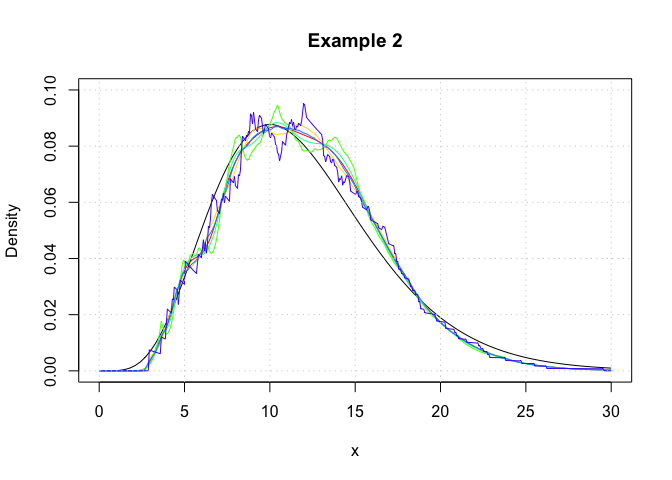### Example 3

In this example, we show that `logdensity` and `logdensity_ftt` yield nearly identical results. Here, log-transformed kernel density estimators are constructed using the `logdensity_ftt` function.

``````## Load 'logKDE' library.
library(logKDE)

## Set a random seed.
set.seed(1)

## Generate strictly positive data.
## Data are generated from a chi-squared distribution with 12 degrees of freedom.
x <- rchisq(100,12)

## Construct a log-KDE using the data, and using each of the available kernel functions.
logKDE1 <- logdensity_fft(x,kernel = 'gaussian',from = 1e-6,to = 30)
logKDE2 <- logdensity_fft(x,kernel = 'epanechnikov',from = 1e-6,to = 30)
logKDE3 <- logdensity_fft(x,kernel = 'laplace',from = 1e-6,to = 30)
logKDE4 <- logdensity_fft(x,kernel = 'logistic',from = 1e-6,to = 30)
logKDE5 <- logdensity_fft(x,kernel = 'triangular',from = 1e-6,to = 30)
logKDE6 <- logdensity_fft(x,kernel = 'uniform',from = 1e-6,to = 30)

## Plot the true probability density function of the generative model.
plot(c(0,30),c(0,0.1),type='n',xlab='x',ylab='Density',main='Example 3')
curve(dchisq(x,12),from = 0,to = 30,add = T)

## Plot each of the log-KDE functions, each in a different rainbow() colour.
lines(logKDE1\$x,logKDE1\$y,col = rainbow(7))
lines(logKDE2\$x,logKDE2\$y,col = rainbow(7))
lines(logKDE3\$x,logKDE3\$y,col = rainbow(7))
lines(logKDE4\$x,logKDE4\$y,col = rainbow(7))
lines(logKDE5\$x,logKDE5\$y,col = rainbow(7))
lines(logKDE6\$x,logKDE6\$y,col = rainbow(7))

## Add a grid for a visual guide.
grid()``````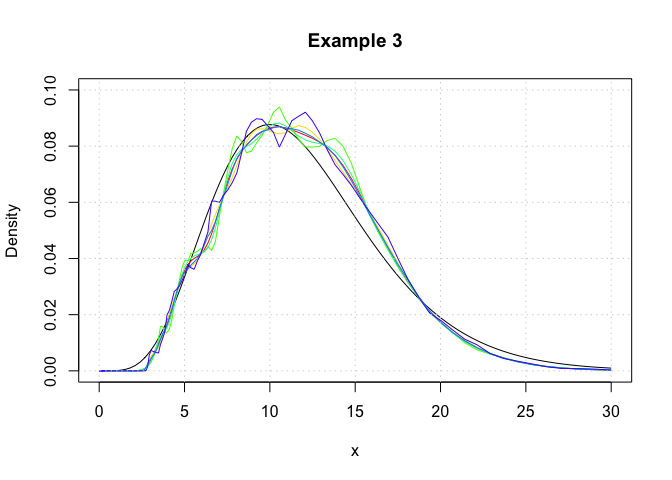We observe that the `logdensity_fft` outputs are noticiably smoother than those of `logdensity`. This is because fast Fourier transformations (FFT) only yield kernel density estimates at discrete points, and the regions between these discrete points are approximated via a linear approximator, namely using the `approx` function. This is the same evaluation technique as that which is used in the function `density`. Additionally the FFT approximation points are evenly space on the real line, whereas those used for `logdensity` are evenly spaced on a log scale.

### Example 4

In this example, we showcase the variety of plugin bandwidth estimators that are available in the package. Here, log-transformed kernel density estimators are constructed using the `logdensity` function.

``````## Load 'logKDE' library.
library(logKDE)

## Set a random seed.
set.seed(1)

## Generate strictly positive data.
## Data are generated from a chi-squared distribution with 12 degrees of freedom.
x <- rchisq(100,12)

## Construct a log-KDE using the data, and using each of the available kernel functions.
logKDE1 <- logdensity(x,bw = 'nrd0',from = 1e-6,to = 30)
logKDE2 <- logdensity(x,bw = 'logcv',from = 1e-6,to = 30)
logKDE3 <- logdensity(x,bw = 'logg',from = 1e-6,to = 30)
logKDE4 <- logdensity(x,bw = 'nrd',from = 1e-6,to = 30)
logKDE5 <- logdensity(x,bw = 'ucv',from = 1e-6,to = 30)
#> Warning in stats::bw.ucv(log(x)): minimum occurred at one end of the range
logKDE6 <- logdensity(x,bw = 'bcv',from = 1e-6,to = 30)
#> Warning in stats::bw.bcv(log(x)): minimum occurred at one end of the range
logKDE7 <- logdensity(x,bw = 'SJ-ste',from = 1e-6,to = 30)
logKDE8 <- logdensity(x,bw = 'SJ-dpi',from = 1e-6,to = 30)

## Plot the true probability density function of the generative model.
plot(c(0,30),c(0,0.1),type='n',xlab='x',ylab='Density',main='Example 4')
curve(dchisq(x,12),from = 0,to = 30,add = T)

## Plot each of the log-KDE functions with different choices of bandwidth, each in a different rainbow() colour.
lines(logKDE1\$x,logKDE1\$y,col = rainbow(9))
lines(logKDE2\$x,logKDE2\$y,col = rainbow(9))
lines(logKDE3\$x,logKDE3\$y,col = rainbow(9))
lines(logKDE4\$x,logKDE4\$y,col = rainbow(9))
lines(logKDE5\$x,logKDE5\$y,col = rainbow(9))
lines(logKDE6\$x,logKDE6\$y,col = rainbow(9))
lines(logKDE7\$x,logKDE7\$y,col = rainbow(9))
lines(logKDE8\$x,logKDE8\$y,col = rainbow(9))

## Add a grid for a visual guide.
grid()``````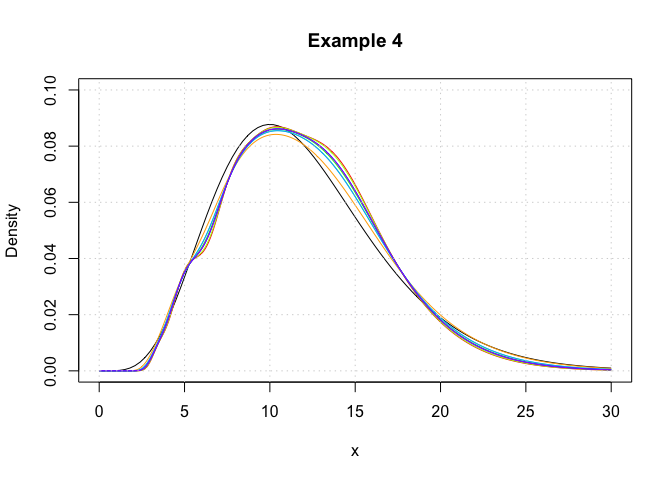## Unit testing

Using the package `testthat`, we have conducted the following unit test for the GitHub build, on the date: 06 August, 2018. The testing files are contained in the tests folder of the respository.

``````

Thank you for your interest in `logKDE`. If you happen to find any bugs in the program, then please report them on the Issues page (https://github.com/andrewthomasjones/logKDE/issues). Support can also be sought on this page. Furthermore, if you would like to make a contribution to the software, then please forward a pull request to the owner of the repository.